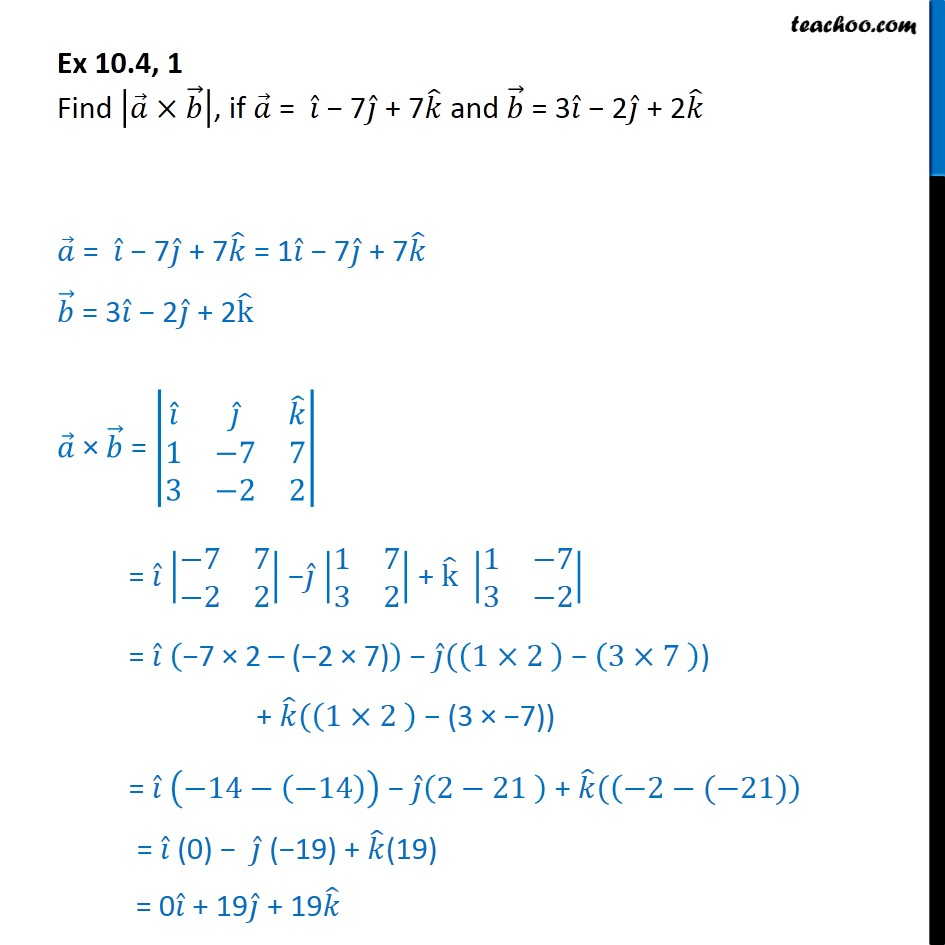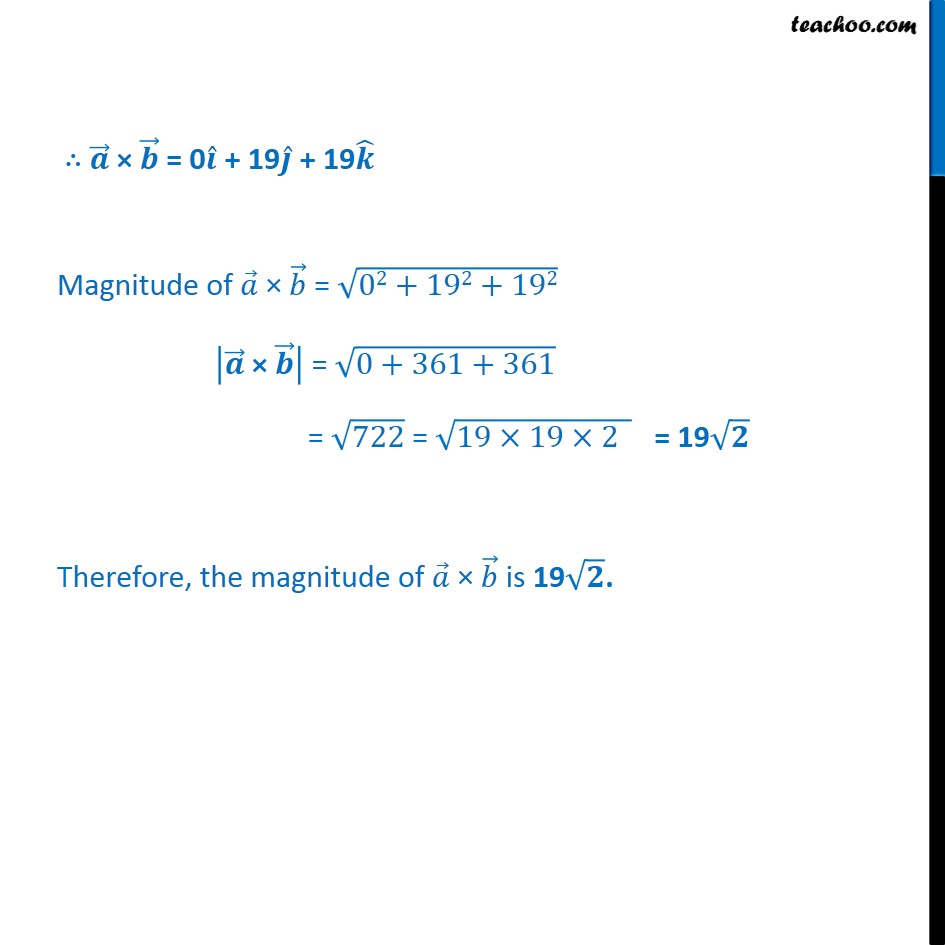Subscribe to our Youtube Channel - https://you.tube/teachoo

1. Chapter 10 Class 12 Vector Algebra
2. Serial order wise
3. Ex 10.4

Transcript

Ex 10.4, 1 Find , if = 7 + 7 and = 3 2 + 2 = 7 + 7 = 1 7 + 7 = 3 2 + 2 k = 1 3 7 2 7 2 = 7 7 2 2 1 7 3 2 + k 1 7 3 2 = 7 2 ( 2 7) ( 1 2 3 7 ) + ( 1 2 (3 7)) = 14 14 2 21 + ( 2 ( 21) = (0) ( 19) + (19) = 0 + 19 + 19 = 0 + 19 + 19 Magnitude of = 02+192+192 = 0+361+361 = 722 = 19 19 2 = 19 Therefore, the magnitude of is 19 .

Ex 10.4